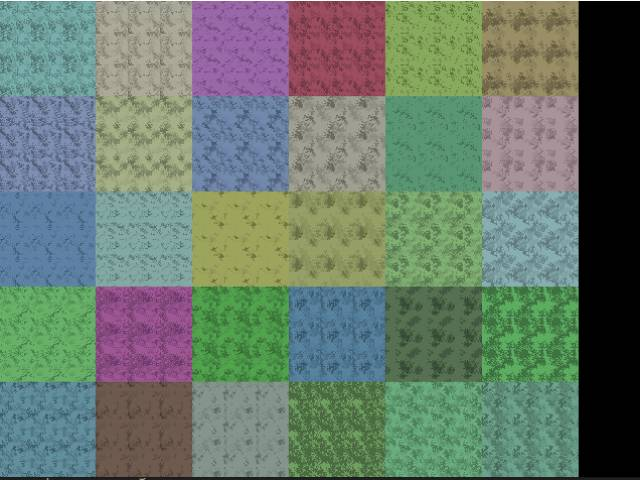-=+=- -=+=- -=+=- -=+=- -=+=- -=+=- -=+=- -=+=- -=+=- -=+=- -=+=- -=+=- -=+=- -=+=- -=+=- -=+=- -=+=- -=+=- -=+=- -=+=- -=+=- -=+=- -=+=- -=+=- -=+=- -=+=- -=+=- -=+=- -=+=- -=+=- (c) WidthPadding Industries 1987 0|494|0 -=+=- -=+=- -=+=- -=+=- -=+=- -=+=- -=+=- -=+=- -=+=- -=+=- -=+=- -=+=- -=+=- -=+=- -=+=- -=+=- -=+=- -=+=- -=+=- -=+=- -=+=- -=+=- -=+=- -=+=- -=+=- -=+=- -=+=- -=+=- -=+=- -=+=-
SoCoder -> Snippet Home -> Generators

PakzCreated : 02 July 2018
Edited : 02 July 2018
Language : Monkey2

### Seamless Grow Tiles(grass/desert etc)

Create Tiles with zones method

Screenshots## Latest Update

This code shows how to create(generate) seamless tiles.

What it does is create spots on a image and grows these. If the spot gets to the edge then the other side is grown onto(seamless part)

There are a couple of variables to get different kind of results. Number of colors, the density(amount of grow) and spread(how many individual starting spots)

I was working on creating a world map tileset and found the code I made for grass tiles useful enough to turn into this example.

In the output on the screen I have drawn 3*3 tiles per tile and the screen filled with other tiles also 3*3..

The code is commented pretty ok'ish I hope.

### Comments

Tuesday, 03 July 2018, 11:41
PakzBtw, a trick I learned is to take a seamless tile and redraw the insides leaving the edges untouched. This creates the same seamless tiles but now a variation of this tile. This for breaking patterns.